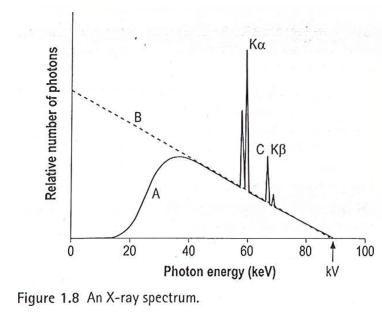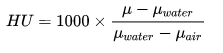# 2017 Medical Physic

2017 Medical Physic SEQ

### A Sketch a labelled graph of the energy spectrum of Tungsten target X ray at 80 kVp. Indicate values of Kɑ, K𝛽 and maximum proton energy ? (20)In the case of tungsten (Z = 74), the standard target material used in medical X-ray tubes,Ek = 70 keV, EL = 12keV, and EM = 2keV  Kɑ = 70-12= 58K𝛽 = 70-2 = 68molybdenum (Z = 42), the target material most commonly used in mammography,Ek = 20 keV and EL = 2.5 keV  Kɑ =17.5K𝛽 ≃ 20

Inherent filtration-

Filtrations occurs due to tube itself - ( light beam diaphragm mirror +  tungsten target + glass cover + insulator oil )

Typically 1 mm aluminium

Filtration  achieved by adding filters to modify the x ray beam - A typical filter for most x-ray tubes is a few mm of aluminum (e.g., ~3 mm)

Totally 2.5 mm aluminium

### C. why filtration is essential(10)

• The x-ray beam emerging from the x-ray tube may contain relatively low energy photons.

• Low-energy photons have a negligible chance of getting through the patient so it  irradiate the patient but add nothing to the image.

• Filters are added to the x-ray tube window to absorb low energy photons.

• This dose reduction is achieved by interposing between the X-ray tube and patient a uniform flat sheet of metal, usually aluminium, and called the added or additional filtration.

• The predominant attenuation process in this filter should be photoelectric absorption.

### d. explain the influence of following in film screen radiography

1.Tube voltage on subject contrast(15)

•  Subject contrast is the difference in x-ray intensity that is transmitted through a lesion in comparison to the adjacent tissues.

• Increasing the x-ray tube voltage (kV) increases the x-ray tube output intensity.

• Relative x-ray beam intensity is proportional to ~kV2

• Increase beam intensity increases the half value layer

• Since the linear attenuation co efficient  = 0.693/HVL, the LAC reduces.

• Increasing the x-ray tube voltage increases the maximum x-ray photon energy

• Accordingly, subject contrast C depends on:

• the thickness t of the structure

• the difference in linear attenuation coefficients,

• At high voltage (kV), there is less absorption due to the less  photoelectric effect and high Compton scatter.

• The higher the kilovoltage (kV), the smaller are the attenuation coefficients and the difference between them and thus the less the contrast

2. line focus principle on image quality(15)• The target is tilted. So ‘actual focal spot’ is  larger than effective focal spot size

• The actual focal spot is the area over which heat is produced and which determines the tube rating.

• Because the target is tilted, the ‘effective focal spot’  is shortened in one direction. This technique produces a small effective  focal spot which reduced geometric unsharpness

• This helps to increase spatial resolution of the image.

3. Heel effect on beam intensity(15))

• Due to anode tilt  X-rays produced within the anode must pass through a portion of the target and are therefore attenuated on their way out of the target

• so attenuation is greater in the anode direction than in the cathode direction because of differences in the path length within the target.

• This results in higher x-ray intensity at the cathode end and lower x-ray intensity at the anode end of the beam.

• This is applied in mammography where  patient is positioned in the cathode side.

4. beam collimation on patient radiation dose(15)

• Collimators adjust the size and shape of the x-ray feld emerging from the tube port

• Maximum beam size should be restricted to the maximum image size required

• Collimation reduces the total patient mass irradiated and, therefore, reduces scatter

• So this will decrease total patient dose from the primary beam and from scatters.

### 2. Explain - in helical ct

1. Slip ring technology      (25)
• A slip ring is electromechanical technology that enables the transmission of power and electrical signals from a stationary to a rotating structure.

• This transmission of power/data is made possible through electrical connections made by stationary brushes pressing against rotating circular conductors.

• CT Slip ring technology was introduced to enable helical (continuous rotating) scanning. Prior to the introduction of Slip Rings, only Axial scanning was possible (which had the need to stop / reverse direction of rotation, after no more than 700 degrees rotation due to the finite length of the attached cables.)

• Slip ring technology eliminated the  need for cables and enabled the continuous rotation of the gantry components.

2. interpolation of data   (25)

• Interpolation refers to the mapping of an image of one matrix size to a display of another size

• Helical CT scanners collect data while x-ray source travelling along a helical path relative to the patient

• CT image reconstruction algorithms assume that the x-ray source has travelled a circular path

• 360° or 180° Interpolation is done to obtain a planer data set from helical data set

360° Interpolation

• Easier

• Resolution along Z direction is poor

180° Interpolation

• Difficult to sort the data

• Resolution along Z direction is better

### b. define CT number, explain why the linear attenuation coefficients are converted to CT numbers (25)

–CT images are maps of the relative linear attenuation values of tissues.

–The relative attenuation coefficient (μ) is normally expressed as CT numbers.

–CT numbers are known as Hounsfield units (HU).

–The HU of material x is HUwhere μ is the attenuation coefficient of the material x and μwater is the attenuation coefficient of water.

–By definition, the HU value for water is always 0.

–The HU value for air is –1,000 since μair is negligible compared to μwater.

CT number CT(x, y) in each pixel at (x, y) of the image is derived from

• CT image reconstruction algorithms assume that the x-ray source has travelled a circular path

• CT reconstruction process results in a 2D matrix of floating point numbers in the range 0.0 to 1.0

• These numbers correspond to the linear attenuation coefficient of the tissues contained in each voxel

• Floating point numbers need more memory than integers

•  CT images are normalized and truncated to integer values (12 bit, 4096 values) between -1000 and 3095

• CT numbers are rescaled linear attenuation coefficients

Since CT use high kVp techniques, CT numbers and CT images derive their contrast mainly from physical properties of tissue that influence Compton scatter

Linear attenuation coefficient varies linearly with density of tissue

CT numbers are quantitative in terms of linear dimensions and can be used to measure tumor volume or lesion diameter

### c explain how windowing technique is used to improve the contrast of CT image (25)

• Windowing is a common technique in digital imaging  to bring out the hidden detail in the image

• Window width and level optimize the appearance of CT images by determining the contrast and brightness levels assigned to the CT image data.

• CT images with a window width of 100 HU and a window level (center) of 50

• HU have HU <0 black, HU >100 white, and HU ~50 mid-gray.

• Window (width and level) settings affect only the displayed image, not the reconstructed image data stored in the computer.